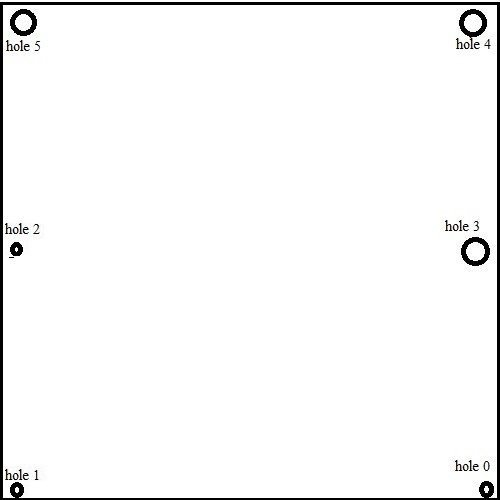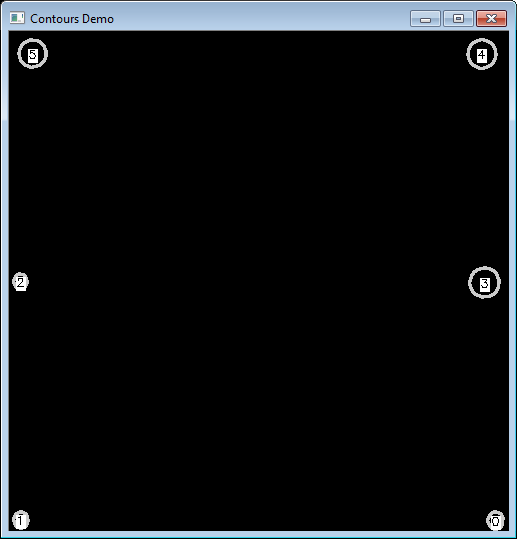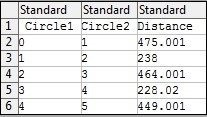## Machine Vision: Measure distance between two objects in an image using OpenCV

January 8, 2018

prototechsolutions

0

### Machine Vision: Measure distance between two objects in an image using OpenCV

Introduction:
OpenCV (i.e. Open Source Computer Vision) is an open source library. It supports C, C ++, Python, and Java. It can be used with multimedia processing using different algorithms like Image Processing, Edge Detection, Object Tracking, etc available in OpenCV.

OpenCV is mainly used in applications like Video Processing, Object Detection, Gesture Recognition, like – to follow Eye Movement, Face Recognition etc. OpenCV can also be used in AR projects to track moving objects, track moving camera etc.

How to calculate the distance between two objects in an image:
Here we are simply measuring the distance between two holes provided in an image using C++. Following is an input image containing holes which are placed at certain distances.Fig 1: Input image containing 6 holes

Let’s give them names from 0 to 5. We will call them as ‘circle’ just for easy understanding. So there are total 6 circles present in an image and we will calculate the distance between a center of the 0th and 1st circle, 1st and 2nd circle, 2nd and 3rd circle, and so on.

Some terms we have used are,

1. Contour: Contours are the outer shape of an object which is used for shape analysis.
2. Canny Edge detection: It is Edge Detection algorithm developed by John F. Canny. In our case, we are finding counter using edges detected by the Canny algorithm.

Following are basic steps which help to find the contour and distance between two points:

Step 1: Import an image and convert imported image from existing color space to Gray, as contours are easy to find in Black-and-white image

cvtColor(img, img_gray, CV_GRAY2BGR);

Find the contours and edges of objects using Canny edge detector which produces edge map,

Canny(img_gray, img_canny, 80, 120);
findContours(img_canny,contours,hierarchy,CV_RETR_EXTERNAL,CV_CHAIN_APPROX_SIMPLE);

Step 2: Initialize a new drawing/image and draw the contours of the image using drawContours().
The arguments in this drawContours() function are as follow:

1. source image
2. contours list
3. Index of contours, index should be -1 to draw all the contours
4. Remaining arguments are color, thickness, etc…

Mat drawing = Mat::zeros( img_canny.size(), CV_8UC1 );
for( int i = 0; i< contours.size(); i++ )
{

drawContours( drawing, contours, i,  color, 2, 8, hierarchy, 0, Point() );
setLabel(drawing,to_string(i),contours[i]);
};

setLabel() is a function used to display text in the center of a contour which provides the label to each circle. These labels will act as an ID for each contour while calculating the distance between contours.

Step 3: Show new drawing/image with contours.

imshow( “Contours Demo”, drawing );

Fig.2 shows counters of the input image with their respective labels at the center of it.

Step 4: Find the distance (in pixels) between centers of contours and write these into a CSV file

for( int i = 0; i< contours.size()-1; i++ )
{
double dist = GetDistance(centers[i].x,centers[i].y,centers[i+1].x,centers[i+1].y);    myfile << i << “, “<< i+1 << “,”<< dist<<endl;
};

We can cross check these values using manually measuring the distances between these labels on a printed image.

Fig.3 is an output obtained in CSV file containing distance (in pixels) between circles present in an input image.

Contour output:Fig 2: Counters with their respective labels at center of it, added programmatically

Output of CSV file:Fig.3: Distance (in pixels) calculated between circles present in contour output

Deep learning based edge detection in OpenCV which is more accurate than the widely popular canny opencv edge detection.

Author: Pranali S